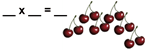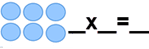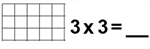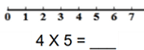# 3rd Grade Math Worksheets: Multiplication

## Multiplication worksheets and tables

Our grade 3 multiplication worksheets start with the meaning of multiplication and follow up with lots of multiplication practice and the multiplication tables; exercises also include multiplying by whole tens and whole hundreds and some column form multiplication. Missing factor questions are also included.

## Meaning of multiplication

Meaning of multiplication sentencesMultiplication sentences and arraysMultiply with arrays

Arrays - draw & multiplyMultiply using a number line## Multiplication facts

Multiplication tables of 2 and 3 2 x 4 =
Multiplication tables of 5 and 10 5 x 3 =
Multiplication tables of 4 and 6 6 x 4 =
Multiplication tables of 7 and 8 8 x 5 =
Multiplication tables of 9 and 3 9 x 3 =
Multiplication tables of 7, 8, and 9 7 x 8 =

Tables 2-5 practice
Tables 6-9 practice
Tables 2-10 practice
Tables 2-12 practice
Tables (full) practice

Multiplication tables 2-10, missing factor 2 x __ = 20
Multiplication tables 2-12, missing factor __ x 12 = 36

## Multiplying by multiples of 10

Multiply 1-digit by whole tens 3 x 10 =
Multiply 1-digit by whole hundreds 4 x 100 =
Multiply whole tens by whole tens 10 x 50 =
Multiply whole tens, missing factor 80 x ___ = 6,400

## Multiply in Columns

2-digit by 1-digit   35
x  8

3-digit by 1-digit 234
x  4

4-digit by 1-digit 1,652
x     7

## Multiplication word problems